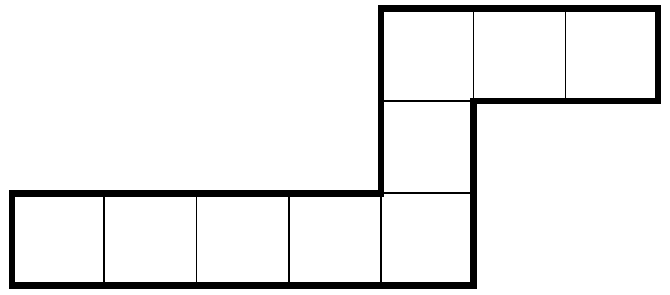#### USAMTS

###### back to index

Find, with proof, all pairs of positive integers $(n, d)$ with the following property: for every integer $S$, there exists a unique non-decreasing sequence of n integers $a_1$, $a_2$, $\cdots$, $a_n$ such that $a_1 + a_2 + \cdots + a_n = S$ and $a_n-a_1 = d$.

Acute scalene triangle $\triangle{ABC}$ has circumcenter $O$ and orthocenter $H$. Points $X$ and $Y$, distinct from $B$ and $C$, lie on the circumcircle of $\triangle{ABC}$ such that $\angle{BXH} = \angle{CYH} = 90^{\circ}$ . Show that if lines $XY$, $AH$, and $BC$ are concurrent, then $OH$ is parallel to $BC$.

Find, with proof, all ordered pairs of positive integers $(a, b)$ with the following property: there exist positive integers $r$, $s$, and $t$ such that for all $n$ for which both sides are defined, $$\binom{\binom{n}{a}}{b}=r\binom{n+s}{t}$$

Lizzie writes a list of fractions as follows. First, she writes $\frac{1}{1}$ , the only fraction whose numerator and denominator add to $2$. Then she writes the two fractions whose numerator and denominator add to $3$, in increasing order of denominator. Then she writes the three fractions whose numerator and denominator sum to 4 in increasing order of denominator. She continues in this way until she has written all the fractions whose numerator and denominator sum to at most $1000$. So Lizzie’s list looks like: $$\frac{1}{1}, \frac{2}{1} , \frac{1}{2} , \frac{3}{1} , \frac{2}{2}, \frac{1}{3}, \frac{4}{1}, \frac{3}{2} , \frac{2}{3}, \frac{1}{4} ,\cdots, \frac{1}{999}$$

Let $p_k$ be the product of the first $k$ fractions in Lizzie’s list. Find, with proof, the value of $p_1 + p_2 +\cdots+ p_{499500}$.

Cyclic quadrilateral $ABCD$ has $AC\perp BD$, $AB + CD = 12$, and $BC + AD = 13$. Find the greatest possible area for $ABCD$.

An eel is a polyomino formed by a path of unit squares that makes two turns in opposite directions. (Note that this means the smallest eel has four cells.) For example, the polyomino shown at right is an eel. What is the maximum area of a $1000\times 1000$ grid of unit squares that can be covered by eels without overlap?